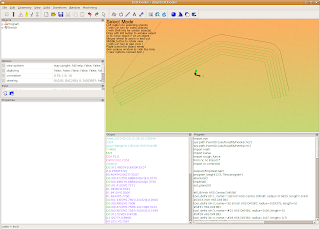## Thursday, May 20, 2010

### Running Python Scripts with the 'Program' Window

HeeksCNC has a unique feature that I really like: all operations create python code that gets processed to make G-code. In the 'Window' menu check the 'Program' option. While you are creating operations and G-code, look at what is happening in the 'Program' window.You can manipulate the python code in the Program window to do all sorts of interesting things. I think that this feature in HeeksCNC gives it a lot of power, similar to APT360. I am very familiar with APT programming and like it a lot, but I really prefer python and the HeeksCNC approach.

The code from the Program window can be edited and used in the window itself. Simply edit the code and then press the 'Py+Go' (Run Python Script) button. Don't just press the 'Go' button, because it will populate the Program window with the normal python code that comes from the operations in HeeksCNC.

You can also copy and edit the python code in your favorite text editor, if you like. I do that because it's what I am familiar with.

The python code can also be run in a stand alone mode- outside of HeeksCNC, if you are careful to fix up any path problems. I sometimes run the python scripts this way, if I have my geometry set and just want to try different variables out with the code, ie different depths of cut.

One simple thing that you can do with the code is to use an operation over and over again with different size tool diameters or different depths. 'if' or 'while' loops make this sort of thing pretty easy to deal with.
In the next screen shot, I took the kurve_funcs.py module and copied/edited it and saved it as rough_funcs.py (I commented out #rapid(z = mat_depth + incremental_rapid_height) on line 229). I simply wanted to keep the tool down in the work for my next trick:Here is my python code that I pasted into the Program window:

import sys
sys.path.insert(0,'/usr/local/lib/heekscnc/')
sys.path.insert(0,'/usr/local/lib/heekscnc')
import math
import kurve
import rough_funcs
from nc.nc import *
import nc.centroid1

output('/tmp/test.tap')
program_begin(123, 'Test program')
absolute()
imperial()
set_plane(0)

#(1/8 inch HSS Centre Drill Bit)
tool_defn( id=1, name='1/8 inch HSS Centre Drill Bit', radius=0.0625, length=0.94)
#(3/16 inch HSS Drill Bit)
tool_defn( id=2, name='3/16 inch HSS Drill Bit', radius=0.09375, length=5)
#(#31 HSS Drill Bit)
tool_defn( id=3, name='#31 HSS Drill Bit', radius=0.06, length=5)
#(#28 HSS Drill Bit)
tool_defn( id=4, name='#28 HSS Drill Bit', radius=0.07, length=0.7)
#(1/8 inch HSS End Mill)
tool_defn( id=5, name='1/8 inch HSS End Mill', radius=0.0625, length=2.5)
#(1/16 inch Carbide End Mill)
tool_defn( id=6, name='1/16 inch Carbide End Mill', radius=0.03125, length=0.3125)
#(#21 HSS Drill Bit)
tool_defn( id=7, name='#21 HSS Drill Bit', radius=0.0795, length=5)
#(engraving cutter)
tool_defn( id=20, name='engraving cutter', radius=0.0625, length=0.625)
comment('G54')
workplane(1)
comment('tool change to 1/8 inch HSS End Mill')
tool_change( id=5)
spindle(7000)
feedrate_hv(33.07086614, 3.937007874)
flush_nc()
clearance = float(0.1968503937)
rapid_down_to_height = float(0.07874015748)
start_depth = float(0)
step_down = float(0.25)
final_depth = float(-0.25)
roll_on = 'auto'
roll_off = 'auto'
tag = None

roll_radius = float(0)
offset_extra = 0
comment('Sketch')
k1 = kurve.new()
kurve.add_point(k1, 0, -1.732283465, -0.7086614173, 0.0, 0.0)
kurve.add_point(k1, 0, 0.157480315, 0.9448818898, 0.0, 0.0)
kurve.add_point(k1, -1, 0.4724409449, 1.062992126, 0.4718257874, 0.5856299213)
kurve.add_point(k1, 0, 1.417322835, 1.102362205, 0.0, 0.0)
kurve.add_point(k1, -1, 1.732283465, 0.9448818898, 1.432465172, 0.7389460933)
kurve.add_point(k1, 0, 2.125984252, 0.07874015748, 0.0, 0.0)

k2 = kurve.new()
kurve.add_point(k2, 0, 2.125984252, 0.07874015748, 0.0, 0.0)
kurve.add_point(k2, 0, 1.732283465, 0.9448818898, 0.0, 0.0)
kurve.add_point(k2, 1, 1.417322835, 1.102362205, 1.432465172, 0.7389460933)
kurve.add_point(k2, 0, 0.4724409449, 1.062992126, 0.0, 0.0)
kurve.add_point(k2, 1, 0.157480315, 0.9448818898, 0.4718257874, 0.5856299213)
kurve.add_point(k2, 0, -1.732283465, -0.7086614173, 0.0, 0.0)

tool_diameter = float(0.75)
rough_funcs.profile(k1, 'right', tool_diameter/2, offset_extra, roll_radius, roll_on, roll_off, tag, rapid_down_to_height, start_depth, step_down, final_depth)

tool_diameter = float(0.625)
rough_funcs.profile(k2, 'left', tool_diameter/2, offset_extra, roll_radius, roll_on, roll_off, tag, rapid_down_to_height, start_depth, step_down, final_depth)

tool_diameter = float(0.5)
rough_funcs.profile(k1, 'right', tool_diameter/2, offset_extra, roll_radius, roll_on, roll_off, tag, rapid_down_to_height, start_depth, step_down, final_depth)

tool_diameter = float(0.375)
rough_funcs.profile(k2, 'left', tool_diameter/2, offset_extra, roll_radius, roll_on, roll_off, tag, rapid_down_to_height, start_depth, step_down, final_depth)

tool_diameter = float(0.25)
rough_funcs.profile(k1, 'right', tool_diameter/2, offset_extra, roll_radius, roll_on, roll_off, tag, rapid_down_to_height, start_depth, step_down, final_depth)

tool_diameter = float(0.125)
rough_funcs.profile(k2, 'left', tool_diameter/2, offset_extra, roll_radius, roll_on, roll_off, tag, rapid_down_to_height, start_depth, step_down, final_depth)

rapid(z = clearance)

program_end()